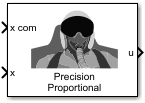# Precision Pilot Model

Represent precision pilot model

• Library:
• Aerospace Blockset / Pilot Models

•## Description

The Precision Pilot Model block represents the pilot model described in Mathematical Models of Human Pilot Behavior . This pilot model is a single input, single output (SISO) model that represents some aspects of human behavior when controlling aircraft. When modeling human pilot models, use this block for more accuracy than that provided by the Tustin Pilot Model and Crossover Pilot Model blocks.

This block has non-linear behavior. If you want to linearize the block (for example, with one of the `linmod` functions), you might need to change the Pade approximation order. The Precision Pilot Model block implementation incorporates the Transport Delay block with the Pade order (for linearization) parameter set to `2` by default. To change this value, use the `set_param` function, for example:

`set_param(gcb,'pade','3')`

This block is an extension of the Crossover Pilot Model block. It implements the equation described in Algorithms.

## Ports

### Input

expand all

Signal command that the pilot model controls, specified as a scalar.

Data Types: `double`

Signal that the pilot model controls, specified as a scalar.

Data Types: `double`

### Output

expand all

Aircraft command, returned as a scalar.

Data Types: `double`

## Parameters

expand all

Aircraft dynamics control. The equalizer form changes according to these values. For more information, see . To help you decide, this table lists the options and associated dynamics.

Option (Controlled Element Transfer Function)Transfer Function of Controlled Element (Yc)Transfer Function of Pilot (Yp)
Proportional

`${K}_{c}$`

Rate or velocity

`$\frac{{K}_{c}}{s}$`

1
Acceleration

`$\frac{{K}_{c}}{{s}^{2}}$`

Second order

`$\frac{{K}_{c}{\omega }_{n}{}^{2}}{{s}^{2}+2\zeta {\omega }_{n}s+{\omega }_{n}^{2}}$`

This table defines the variables used in the list of control options.

VariableDescription
K c Aircraft gain.
T I Lag constant.
ζDamping ratio for the aircraft.
ω n Natural frequency of the aircraft.

#### Programmatic Use

 Block Parameter: `sw_popup` Type: character vector Values: `'Proportion'` | `'Rate or velocity'` | `'Acceleration'` | ```'Second order'``` Default: `'Proportion'`

Pilot gain, specified as a double scalar.

#### Programmatic Use

 Block Parameter: `Kp` Type: character vector Values: double scalar Default: `'1'`

Total pilot time delay, specified as a double scalar, in seconds. This value typically ranges from 0.1 s to 0.2 s.

#### Programmatic Use

 Block Parameter: `time_delay` Type: character vector Values: double scalar Default: `'0.1'`

Equalizer lead constant, specified as a double scalar.

#### Dependencies

To enable this parameter, set Type of control to `Proportional`, `Acceleration`, or ```Second order```.

#### Programmatic Use

 Block Parameter: `TL` Type: character vector Values: double scalar Default: `'1'`

Equalizer lag constant, specified as a double scalar.

#### Dependencies

To enable this parameter, set Type of control to `Proportional`, `Acceleration`, or ```Second order```.

#### Programmatic Use

 Block Parameter: `TI` Type: character vector Values: double scalar Default: `'5'`

Neuromuscular system lag constant, specified as a double scalar.

#### Programmatic Use

 Block Parameter: `TN1` Type: character vector Values: double scalar Default: `0.1`

Undamped natural frequency of the neuromuscular system, specified as a double scalar, in rad/s.

#### Programmatic Use

 Block Parameter: `nat_freq` Type: character vector Values: double scalar Default: `20`

Damping neuromuscular system, specified as a double scalar.

#### Programmatic Use

 Block Parameter: `damp` Type: character vector Values: double scalar Default: `0.7`

Controlled element undamped natural frequency, specified as a double scalar, in rad/s.

#### Dependencies

To enable this parameter, set Type of control to `Second order`.

#### Programmatic Use

 Block Parameter: `omega_m` Type: character vector Values: double scalar Default: `15`

## Algorithms

When calculating the model, this block also takes into account the neuromuscular dynamics of the pilot. This block implements the following equation:

`${Y}_{p}={K}_{p}{e}^{-\tau s}\left(\frac{{T}_{L}s+1}{{T}_{I}s+1}\right)\right)\left[\frac{1}{\left({T}_{N1}s+1\right)\left(\frac{{s}^{2}}{{\omega }_{N}{}^{2}}+\frac{2{\zeta }_{N}}{{\omega }_{N}}s+1\right)}\right],$`

where:

VariableDescription
K p Pilot gain.
τ Pilot delay time.
T L Time lead constant for the equalizer term.
T I Time lag constant.
T N1 Time constant for the neuromuscular system.
ω N Undamped frequency for the neuromuscular system.
ζ N Damping ratio for the neuromuscular system.

A sample value for the natural frequency and the damping ratio of a human is 20 rad/s and 0.7, respectively. The term containing the lead-lag term is the equalizer form. This form changes depending on the characteristics of the controlled system. A consistent behavior of the model can occur at different frequency ranges other than the crossover frequency.

 McRuer, D. T., Krendel, E., Mathematical Models of Human Pilot Behavior . Advisory Group on Aerospace Research and Development AGARDograph 188, Jan. 1974.

 McRuer, D. T., Graham, D., Krendel, E., and Reisener, W., Human Pilot Dynamics in Compensatory Systems . Air Force Flight Dynamics Lab. AFFDL-65-15. 1965.

## Support평가판 신청